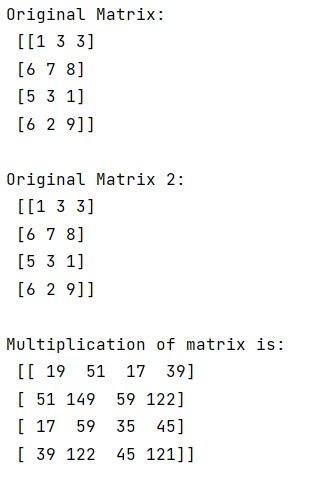# How does multiplication differ for NumPy Matrix vs Array classes?

Learn how to subsample every nth entry in a NumPy array in Python?
Submitted by Pranit Sharma, on December 28, 2022

NumPy is an abbreviated form of Numerical Python. It is used for different types of scientific operations in python. Numpy is a vast library in python which is used for almost every kind of scientific or mathematical operation. It is itself an array which is a collection of various methods and functions for processing the arrays.

The numpy docs recommend using array instead of the matrix for working with matrices. However, (*) does not perform matrix multiplication, which is why we need to use the function for matrix multiplication.

The important points about the NumPy array and NumPy matrix are:

• NumPy matrix is a subclass of the NumPy array
• NumPy array operations are element-wise
• NumPy matrix operations follow the ordinary rules of linear algebra

Note: This method only works unless and until the matrices are not converted to arrays.

Let us understand with the help of an example,

## Python code to demonstrate the example ' how does multiplication differ for NumPy Matrix vs Array classes'

```# Import numpy
import numpy as np

# Import linear algebra module
from numpy import linalg

# Creating a numpy matrix
mat = np.matrix("1 3 3; 6 7 8; 5 3 1; 6 2 9")

# Display original matrix
print("Original Matrix:\n",mat,"\n")

# Creating another numpy matrix
mat2 = np.matrix("1 3 3; 6 7 8; 5 3 1; 6 2 9")

# Display original matrix 2
print("Original Matrix 2:\n",mat2,"\n")

# Finding transpose of mat2 so that a require order of
# matrix can be used for multiplication
t_of_mat2 = mat2.T

# Multiplying mat1 with transpose of mat2
res = mat * t_of_mat2

# Display result
print("Multiplication of matrix is:\n",res,"\n")
```

Output: